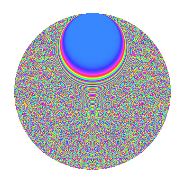# Properties

 Label 3150.2.eoLevel 3150 Weight 2 Character orbit eo Rep. character $$\chi_{3150}(317,\cdot)$$ Character field $$\Q(\zeta_{60})$$ Dimension 3840 Sturm bound 1440

# Learn more about

## Defining parameters

 Level: $$N$$ = $$3150 = 2 \cdot 3^{2} \cdot 5^{2} \cdot 7$$ Weight: $$k$$ = $$2$$ Character orbit: $$[\chi]$$ = 3150.eo (of order $$60$$ and degree $$16$$) Character conductor: $$\operatorname{cond}(\chi)$$ = $$1575$$ Character field: $$\Q(\zeta_{60})$$ Sturm bound: $$1440$$

## Dimensions

The following table gives the dimensions of various subspaces of $$M_{2}(3150, [\chi])$$.

Total New Old
Modular forms 11648 3840 7808
Cusp forms 11392 3840 7552
Eisenstein series 256 0 256

## Trace form

 $$3840q$$ $$\mathstrut +\mathstrut O(q^{10})$$ $$3840q$$ $$\mathstrut -\mathstrut 12q^{15}$$ $$\mathstrut -\mathstrut 480q^{16}$$ $$\mathstrut -\mathstrut 36q^{17}$$ $$\mathstrut -\mathstrut 16q^{18}$$ $$\mathstrut -\mathstrut 36q^{27}$$ $$\mathstrut +\mathstrut 12q^{30}$$ $$\mathstrut +\mathstrut 20q^{33}$$ $$\mathstrut +\mathstrut 40q^{39}$$ $$\mathstrut +\mathstrut 4q^{42}$$ $$\mathstrut +\mathstrut 140q^{45}$$ $$\mathstrut +\mathstrut 48q^{50}$$ $$\mathstrut -\mathstrut 32q^{57}$$ $$\mathstrut -\mathstrut 24q^{58}$$ $$\mathstrut -\mathstrut 8q^{60}$$ $$\mathstrut +\mathstrut 228q^{63}$$ $$\mathstrut -\mathstrut 24q^{65}$$ $$\mathstrut +\mathstrut 140q^{69}$$ $$\mathstrut -\mathstrut 8q^{72}$$ $$\mathstrut -\mathstrut 232q^{75}$$ $$\mathstrut +\mathstrut 48q^{77}$$ $$\mathstrut +\mathstrut 16q^{78}$$ $$\mathstrut +\mathstrut 120q^{84}$$ $$\mathstrut -\mathstrut 40q^{87}$$ $$\mathstrut +\mathstrut 300q^{89}$$ $$\mathstrut +\mathstrut 80q^{90}$$ $$\mathstrut +\mathstrut 24q^{92}$$ $$\mathstrut +\mathstrut 12q^{93}$$ $$\mathstrut +\mathstrut O(q^{100})$$

## Decomposition of $$S_{2}^{\mathrm{new}}(3150, [\chi])$$ into irreducible Hecke orbits

The newforms in this space have not yet been added to the LMFDB.

## Decomposition of $$S_{2}^{\mathrm{old}}(3150, [\chi])$$ into lower level spaces

$$S_{2}^{\mathrm{old}}(3150, [\chi]) \cong$$ $$S_{2}^{\mathrm{new}}(1575, [\chi])$$$$^{\oplus 2}$$